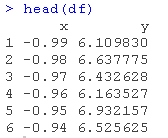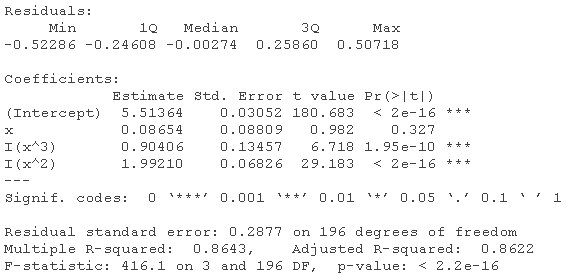1. 准备数据
2. 拟合模型
3. 寻找最佳拟合
4. 源代码

# 准备数据

function(x) x^3+2*x^2+5

df = data.frame(x = x, y = y)
head(df)plot(df$x, df$y

# 拟合模型pred = predict(model,data=df)

# 寻找最佳拟合

 lines(df\$x, predict(lm(y~x, data=df)), type="l"  lwd=2)

legend("topleft",
legend = c("y~x,  - 线性","y~x^2", "y~x^3", "y~x^3+x^2"),

1. 用plot()函数作图。

# R语言里的非线性模型：多项式回归、局部样条、平滑样条、 广义相加模型GAM分析

2. 用ggplot()作图。

ggplot(data=df ) +
geom_smooth(  y~I(x^3)+I(x^2))

### 随时关注您喜欢的主题

Kaizong Ye拓端研究室（TRL）的研究员。

​非常感谢您阅读本文，如需帮助请联系我们！QQ在线咨询

15121130882

0571-63341498

## 关注有关新文章的微信公众号

This will close in 0 seconds